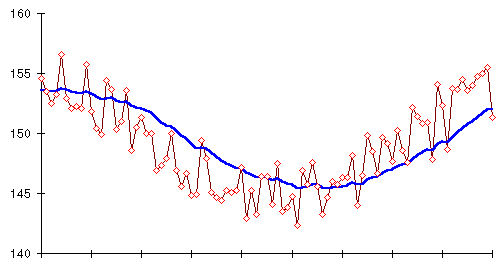Next: The truth in Up: Moving averages Previous: Weighted moving averages

### Exponentially smoothed weighted moving Marvin

Replacing the simple moving average with an exponentially smoothed one with a smoothing constant of 0.9 (roughly equivalent to a 20 day simple moving average in terms of lagging the trend), we get the following plot showing the trend line produced by the moving average versus the original daily weights from which it was calculated.At last we've found a tool that accurately extracts the trend of actual body weight, the direct consequence of the balance between what goes in to the rubber bag and what gets burned, from all the distraction and confusion based on whatever's in the bag at the moment you step on the scale. Now we'll learn how to interpret this trend line.

By John Walker